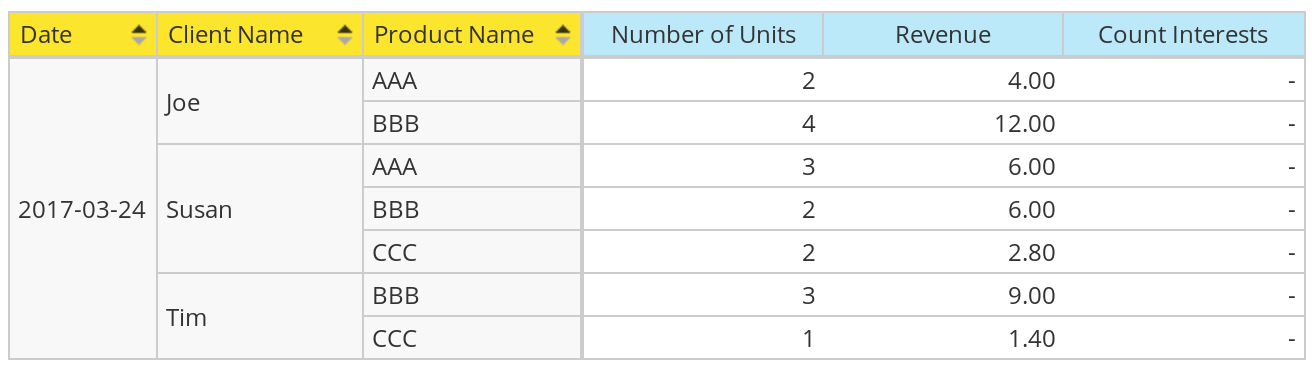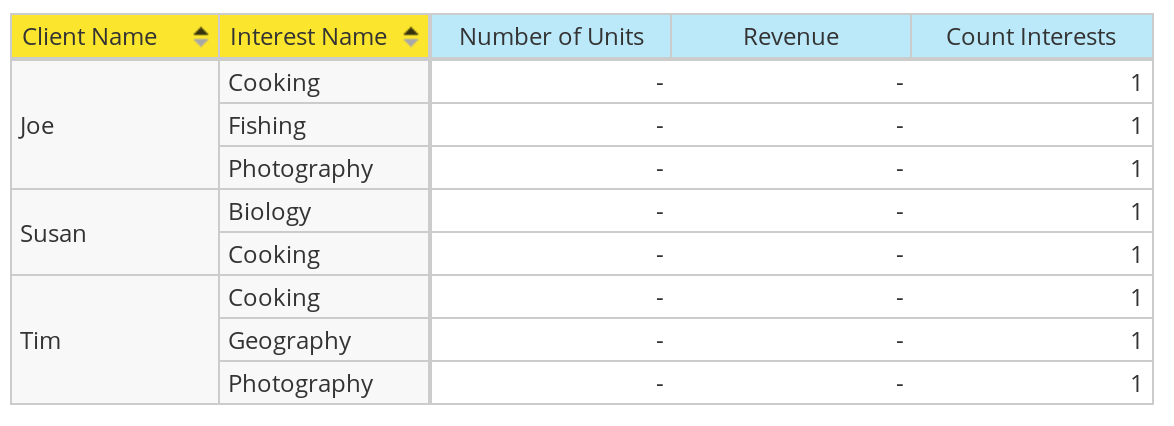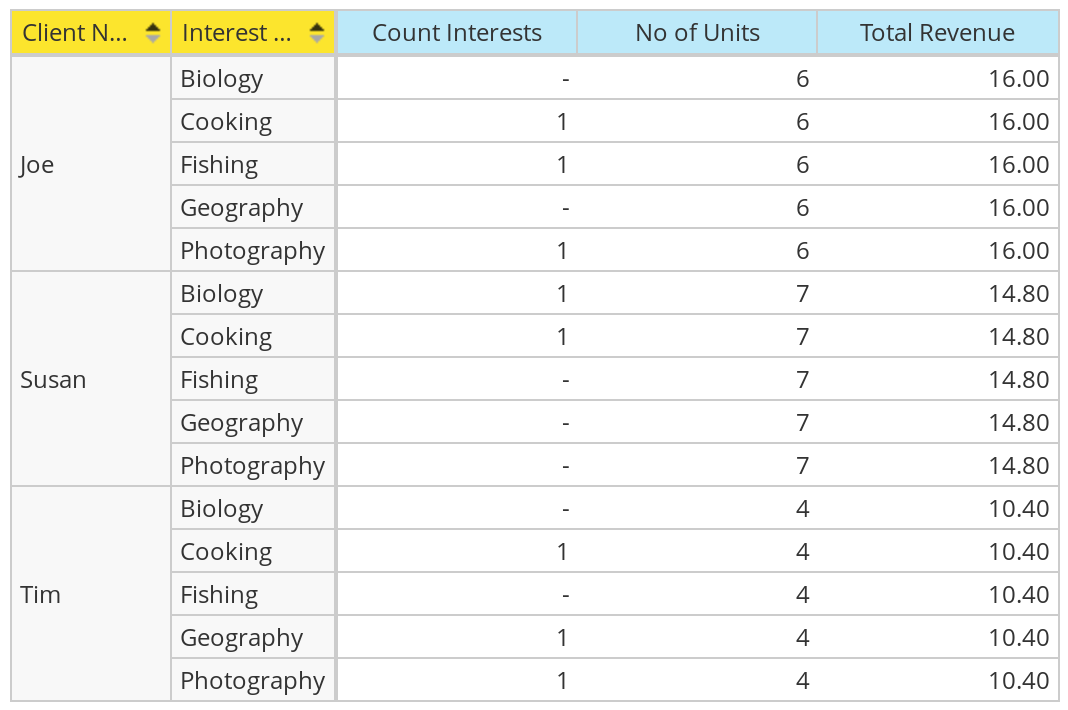Mondrian: Modeling a Multivalued Dimension Attribute

20 Mar 2017

# AGGREGATION TYPE: AVERAGE

A vivid reader called Bruno recently contacted me after reading my article on bridge tables: He was after a solution for a challenging scenario. The task was to create a model for students that receive grades for the courses they attend. A student can have one or more hobbies and one of the analysis questions was: What is the average grade for students with hobby X that attend course Y? This caught my interest and I was eager to find out how this would be implemented with Mondrian.

In our example we use the following data:

• Student Bob attended the Maths course and scored a 7 (grade). He also attended the Physics course and scored a 3 (grade). Bob’s hobbies are Gaming and Reading.
• Student Lilian attended the Maths course and scored a 8 (grade). Her hobbies are Gaming, Jogging and Writing.

While I had some ideas only a talk with Nelson Sousa led to a viable solution: He suggested creating two separate fact tables, one which stores the grades per student per course (naturally you would have a date as well, but we ignore it for simplicity sake). And another fact table which just stores the student and hobbies relationship. The latter one does not necessarily have to have a measure in the fact table itself, however, I added one for completeness sake (a constant of value `1`). And finally there is a student dimension serving as the link between the two fact tables:

`multivalued.dim_student`:

`student_tk` `student_name`
1 Bob
2 Lilian

`multivalued.fact_grades`:

`student_tk` `course_name` `grade`
1 Math 7
1 Math 4
1 Physics 3
2 Math 8

`multivalued.fact_student_hobbies`:

`student_tk` `hobby_name` `cnt`
1 Gaming 1
2 Gaming 1
2 Jogging 1
2 Writing 1

## Create standard Cubes to answer simple Questions

The important point here is that we want to employ an aggregate function of type Average for grades (since grades cannot be summed):

Cube Definition:

``````<Schema name="Multivalued Dimension Attribute">
<Dimension type="StandardDimension" name="Student">
<Hierarchy name="Student Name" hasAll="true" primaryKey="student_tk">
<Table name="dim_student" schema="multivalued"/>
<Level name="Student Name" column="student_name" type="String" uniqueMembers="true" levelType="Regular"/>
</Hierarchy>
</Dimension>
<Dimension type="TimeDimension" name="Date">
<Hierarchy name="Date" hasAll="true" primaryKey="date_tk">
<Table name="dim_date" schema="multivalued"/>
<Level name="Date" column="the_date" type="Date" uniqueMembers="true" levelType="TimeDays"/>
</Hierarchy>
</Dimension>
<Dimension type="StandardDimension" name="Course Name">
<Hierarchy name="Course Name" hasAll="true">
<Level name="Course Name" column="course_name" type="String" uniqueMembers="false" levelType="Regular">
</Level>
</Hierarchy>
</Dimension>
<DimensionUsage source="Student" name="Student" foreignKey="student_tk"/>
</Cube>
<Cube name="Hobbies" cache="true" enabled="true">
<Table name="fact_student_hobbies" schema="multivalued"/>
<Dimension type="StandardDimension" name="Hobby Name">
<Hierarchy name="Hobby Name" hasAll="true">
<Level name="Hobby Name" column="hobby_name" type="String" uniqueMembers="false" levelType="Regular">
</Level>
</Hierarchy>
</Dimension>
<DimensionUsage source="Student" name="Student" foreignKey="student_tk"/>
<Measure name="Count Hobbies" column="cnt" datatype="Integer" formatString="#,###" aggregator="sum"/>
</Cube>
</Schema>
``````

We created a cube for each of the fact tables to answer specific questions:

Q: What is the average grade by student?

``````SELECT
[Student.Student Name].Children ON ROWS
``````

Result:

Bob 4.667
Lilian 8

Q: What is the average grade by course?

``````SELECT
[Course Name].Children ON ROWS
``````

Result:

Math 6.333
Physics 3

Q: How many students have Gaming as a Hobby?

``````SELECT
NON EMPTY [Student.Student Name].Members ON ROWS
, [Measures].[Count Hobbies] ON COLUMNS
FROM [Hobbies]
WHERE
[Hobby Name].[Gaming]
``````

Result:

Student Name Count Hobbies
All Student.Student Names 2
Bob 1
Lilian 1

## Create a Virtual Cube to answer complex Questions

And then we can create a virtual cube to answer questions that span both fact tables. We call this cube Grades and Hobbies.

I recommend reading through the official Mondrian Docu on Virtual Cubes to understand how to construct virtual cubes.

### Virtual Cube: How to join the base cubes

Use a global (conforming) dimension for this purpose. In our case the `Student` dimension links the two fact tables and is defined as global dimension.

``````<VirtualCubeDimension name="Student"/>
``````

Important: Note that with the common dimension we do not define the `cubeName` attribute value.

Q: Does this then in effect require the definition of `CubeUsage` as well since in this case the `VirtualCubeDimension` element does not specify a value for the `cubeName`? Otherwise how does Mondrian know that this global dimension exists in both base cubes and that it can hence use it to join the base cubes?

A: `CubeUsage` is optional and not required. If you reference a global dimension in the virtual cube, Mondrian will check the base cubes defined for the virtual measures to see if this global dimension is referenced in the base cubes.

Full Virtual Cube definition:

``````<VirtualCube name="Grades and Hobbies" enabled="true">
<!-- common dimensions -->
<VirtualCubeDimension name="Student"/>
<!-- specific dimensions -->
<VirtualCubeDimension name="Hobby Name" cubeName="Hobbies"/>
<VirtualCubeMeasure name="[Measures].[Count Hobbies]" cubeName="Hobbies"/>
</VirtualCube>
``````

Let’s create a list of students with a count of hobbies and the average of grades:

``````SELECT
[Student.Student Name].Children ON ROWS
, {[Measures].[Grade], [Measures].[Count Hobbies]} ON COLUMNS
``````
Bob 4.667 2
Lilian 8 3

The below result shows quite an interesting result: count of hobbies is only available on the All level, but grades are available everywhere:

``````SELECT
[Student.Student Name].Members * [Course Name].Members ON ROWS
, {[Measures].AllMembers} ON COLUMNS
``````
Student Name Course Name Count Hobbies Grade
All Student.Student Names All Course Names 5 5.5
Math   6.333
Physics   3
Bob All Course Names 2 4.667
Math   5.5
Physics   3
Lilian All Course Names 3 8
Math   8
Physics

This makes sense though, as the counts of hobbies can only be available on an `All Courses` level as we do not store them by course!

Let’s get a list of students that have Gaming as a hobby:

``````SELECT
FILTER(
[Student.Student Name].Children
, (
[Hobby Name].[Gaming]
, [Measures].[Count Hobbies]
) > 0
) ON ROWS
, {} ON COLUMNS
``````

Result:

Student Name
Bob
Lilian

Q: What is the average grade by Hobby?

``````SELECT
[Course Name].Members ON ROWS
``````
Math 6.333
Physics 3

Learning Exercise

Let’s go back to understanding some basics: Pay attention to the slicer (`WHERE`) in the following query:

``````WITH SET STUDENTS AS
FILTER(
[Student.Student Name].Children
, (
, [Measures].[Count Hobbies]
) > 0
)
SELECT
STUDENTS ON ROWS
WHERE [Course Name].[Course Name].[Math]
``````

Interestingly enough we do not get any records returned! However, if we move the constrain from the slicer to one of the axis, all is fine:

``````WITH SET STUDENTS AS
FILTER(
[Student.Student Name].Children
, (
, [Measures].[Count Hobbies]
) > 0
)
SELECT
STUDENTS *  [Course Name].[Course Name].[Math] ON ROWS
``````

Result:

Bob Math 5.5

The reason for this is that the slicer also influences calculated members and sets directly, whereas if we move the constrain onto one of axis, calculated members and sets will be evaluated without this constrain.

Or alternatively you can also add the `All` member to the filter to override the constrain in the slicer:

``````WITH SET STUDENTS AS
FILTER(
[Student.Student Name].Children
, (
, [Course Name].[All Course Names]
, [Measures].[Count Hobbies]
) > 0
)
SELECT
STUDENTS ON ROWS
WHERE [Course Name].[Course Name].[Math]
``````

This will produce exactly the same result.

Next let’s look at the original question:

Q: What is the average grade of students that attended the math course and have Gaming as a hobby?

This query returns all students that are interest in Gaming and attended the math course:

``````WITH
SET STUDENTS AS
FILTER(
[Student.Student Name].Children
, (
[Hobby Name].[Gaming]
, [Measures].[Count Hobbies]
) > 0
)
SELECT
STUDENTS *  [Course Name].[Course Name].[Math] ON ROWS
``````
Bob Math 5.5
Lilian Math 8

Let’s try to answer the original question now:

``````WITH
SET STUDENTS AS
FILTER(
[Student.Student Name].Children
, (
[Hobby Name].[Gaming]
, [Course Name].[All Course Names]
, [Measures].[Count Hobbies]
) > 0
)
SELECT
STUDENTS ON ROWS
WHERE [Course Name].[Course Name].[Math]
``````

The trick here is to get a list of students that have a specific hobby before asking for the grades by course. Also, note that we moved the constrain on the Math course into the slicer (`WHERE` clause). Since this influences the calculated set as well, we had to explicitly add `[Course Name].[All Course Names]` there as well.

Result:

Bob 5.5
Lilian 8

# AGGREGATION TYPE: SUM

In this variation of the theme we imagine we are a company selling various merchandise. The clients have various interests and we want to see how much revenue we generate by user interest. We do not want to weight the interests - we allocate all revenue to every single interest. The aim is to find out which interest generates most revenue (well, we won’t quite do this here, but you’ll get the idea).

`multivalued.dim_client`:

`````` client_tk | client_name
-----------+-------------
1 | Joe
2 | Susan
3 | Tim
``````

`multivalued.fact_client_interests`:

`````` client_tk | interest_name | cnt
-----------+---------------+-----
1 | Fishing       |   1
1 | Photography   |   1
1 | Cooking       |   1
2 | Cooking       |   1
2 | Biology       |   1
3 | Geography     |   1
3 | Photography   |   1
3 | Cooking       |   1
``````

`multivalued.dim_product`:

`````` product_tk | product_name | unit_price
------------+--------------+------------
1 | AAA          |       2.00
2 | BBB          |       3.00
3 | CCC          |       1.40
``````

`multivalued.dim_date`:

`````` date_tk  |  the_date
----------+------------
20170324 | 2017-03-24
``````

`multivalued.fact_sales`:

`````` date_tk  | client_tk | product_tk | no_of_units | amount_spent
----------+-----------+------------+-------------+--------------
20170324 |         1 |          1 |           2 |            4
20170324 |         2 |          1 |           3 |            6
20170324 |         1 |          2 |           4 |           12
20170324 |         2 |          2 |           2 |            6
20170324 |         3 |          2 |           3 |            9
20170324 |         2 |          3 |           2 |          2.8
20170324 |         3 |          3 |           1 |          1.4
``````

The cube definition: In this case client is our global dimension which links the two cubes (sales and interests). The setup is straight forward, so I will not go into any details:

``````<Schema name="Multivalued Dimension Attribute">
<Dimension type="TimeDimension" name="Date">
<Hierarchy name="Date" hasAll="true" primaryKey="date_tk">
<Table name="dim_date" schema="multivalued"/>
<Level name="Date" column="the_date" type="Date" uniqueMembers="true" levelType="TimeDays"/>
</Hierarchy>
</Dimension>
<Dimension type="StandardDimension" name="Product">
<Hierarchy name="Product Name" hasAll="true" primaryKey="product_tk">
<Table name="dim_product" schema="multivalued"/>
<Level name="Product Name" column="product_name" type="String" uniqueMembers="true" levelType="Regular"/>
</Hierarchy>
</Dimension>
<Dimension type="StandardDimension" name="Client">
<Hierarchy name="Client Name" hasAll="true" primaryKey="client_tk">
<Table name="dim_client" schema="multivalued"/>
<Level name="Client Name" column="client_name" type="String" uniqueMembers="true" levelType="Regular"/>
</Hierarchy>
</Dimension>
<Cube name="Sales" cache="true" enabled="true">
<Table name="fact_sales" schema="multivalued"/>
<DimensionUsage source="Date" name="Date" foreignKey="date_tk"/>
<DimensionUsage source="Client" name="Client" foreignKey="client_tk"/>
<DimensionUsage source="Product" name="Product" foreignKey="product_tk"/>
<Measure name="Number of Units" column="no_of_units" datatype="Integer" formatString="#,###" aggregator="sum"/>
<Measure name="Revenue" column="amount_spent" datatype="Numeric" formatString="#,###.00" aggregator="sum"/>
</Cube>
<Cube name="Interests" cache="true" enabled="true">
<Table name="fact_client_interests" schema="multivalued"/>
<Dimension type="StandardDimension" name="Interest Name">
<Hierarchy name="Interest Name" hasAll="true">
<Level name="Interest Name" column="interest_name" type="String" uniqueMembers="true" levelType="Regular"/>
</Hierarchy>
</Dimension>
<DimensionUsage source="Client" name="Client" foreignKey="client_tk"/>
<Measure name="Count Interests" column="cnt" datatype="Integer" formatString="#,###" aggregator="sum"/>
</Cube>
<VirtualCube name="Sales and Interests" enabled="true">
<!-- common dimensions -->
<VirtualCubeDimension name="Client"/>
<!-- specific dimensions -->
<VirtualCubeDimension name="Date" cubeName="Sales"/>
<VirtualCubeDimension name="Product" cubeName="Sales"/>
<VirtualCubeDimension name="Interest Name" cubeName="Interests"/>
<VirtualCubeMeasure name="[Measures].[Number of Units]" cubeName="Sales"/>
<VirtualCubeMeasure name="[Measures].[Revenue]" cubeName="Sales"/>
<VirtualCubeMeasure name="[Measures].[Count Interests]" cubeName="Interests"/>
</VirtualCube>
</Schema>
``````

We created a cube for each of the fact tables to answer specific questions:

Q: How much revenue do we generate by product?

``````SELECT
[Product.Product Name].Children ON ROWS
, {[Measures].[Number of Units], [Measures].[Revenue]} ON COLUMNS
FROM [Sales]
``````
Product Name Number of Units Revenue
AAA 5 10.00
BBB 9 27.00
CCC 3 4.20

Q: How much revenue do we generate per user?

``````select
[Client.Client Name].Children ON ROWS
, {[Measures].[Number of Units], [Measures].[Revenue]} ON COLUMNS
FROM [Sales]
``````
Client Name Number of Units Revenue
Joe 6 16.00
Susan 7 14.80
Tim 4 10.40

Q: How many clients are interested in Cooking?

``````SELECT
NON EMPTY [Client.Client Name].Members ON ROWS
, [Measures].[Count Interests] ON COLUMNS
FROM [Interests]
WHERE
[Interest Name].[Cooking]
``````
Client Name Count Interests
All Client.Client Names 3
Joe 1
Susan 1
Tim 1

And we also created a virtual cube to answer questions involving both cubes.

Q: How much did users interesting in Photography spend?

Let’s get the list of relevant clients first:

``````SELECT
FILTER(
[Client.Client Name].Children
, (
[Interest Name].[Interest Name].[Photography]
, [Measures].[Count Interests]
) > 0
) ON ROWS
, {} ON COLUMNS
FROM [Sales and Interests]
``````

And then construct the final query:

``````WITH
SET CLIENTS AS
FILTER(
[Client.Client Name].Children
, (
[Interest Name].[Interest Name].[Photography]
, [Product.Product Name].[All Product.Product Names]
, [Measures].[Count Interests]
) > 0
)
SELECT
CLIENTS ON ROWS
, {[Measures].[Revenue]} ON COLUMNS
FROM [Sales and Interests]
WHERE [Product.Product Name].[Product Name].[AAA]
``````
Client Name Revenue
Joe 4.00
Tim

# Valid Measure

In some cases you might want to show figures even though in the current selection they are not available. Take this senario:

When we choose `Date`, `Client` and `Product Name`we can see values for `Number of Units` and `Revenue`, but not for `Count Interests`:If we want to see the `Count Interests` figures, but have to choose `Client Name` and `Interest` as dimensions, but in this case `Number of Units` and `Revenue` are empty:Now let’s assume we wanted to show the closest `Number of Units` and `Revenue` in this context, how could we implement this. We do know that the closest available figure would be the total by `Client Name`, so basically this figure would be repeated several times. It is always worth confirming with business users if this is indeed an acceptable behaviour.

To implement this, set the original virtual measures to invisible and create a calculated member in the virtual cube using the `ValidMeasure()` functions, which should reference the original virtual measure. It should like this:

``````<VirtualCube name="Sales and Interests" enabled="true">
<!-- common dimensions -->
<VirtualCubeDimension name="Client"/>
<!-- specific dimensions -->
<VirtualCubeDimension name="Date" cubeName="Sales"/>
<VirtualCubeDimension name="Product" cubeName="Sales"/>
<VirtualCubeDimension name="Interest Name" cubeName="Interests"/>
<VirtualCubeMeasure name="[Measures].[Number of Units]" cubeName="Sales" visible="false"/>
<VirtualCubeMeasure name="[Measures].[Revenue]" cubeName="Sales" visible="false"/>
<VirtualCubeMeasure name="[Measures].[Count Interests]" cubeName="Interests"/>
<CalculatedMember name="No of Units" dimension="Measures">
<Formula>
<![CDATA[
ValidMeasure([Measures].[Number of Units])
]]>
</Formula>
</CalculatedMember>
<CalculatedMember name="Total Revenue" dimension="Measures">
<Formula>
<![CDATA[
ValidMeasure([Measures].[Revenue])
]]>
</Formula>
</CalculatedMember>
</VirtualCube>
``````

The report based on this new virtual cube will look like this:Here in example for Joe we know that he is interested in cooking, fishing and photography and that in total he has bought 6 units which resulted in a total revenue of 16.

# Virtual Cubes: Denormalised Fact Tables

Virtual cubes rely on global dimensions, however, if you are dealing with denormalised fact tables, chances are that you use degenerate dimensions. So does this mean you cannot create virtual cubes for them? Not quite. You can trick the system:

Just create a view for each degenerate table (e.g. `vw_dim_location`) and use this one in the table reference of the global dim.